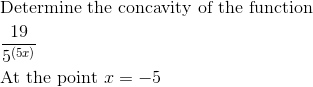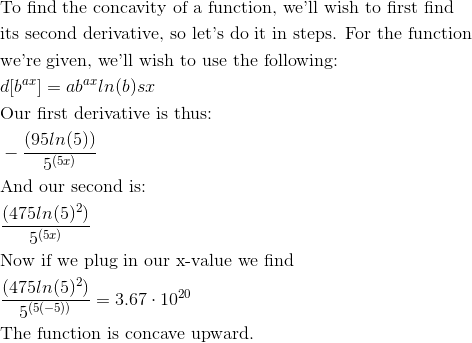# AP Calculus AB : Relationship between the concavity of ƒ and the sign of ƒ''

## Example Questions

← Previous 1 3

### Example Question #1 : Second Derivatives

What is the concavity of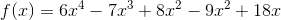at x=1?

Concave Horizontal

No Concavity

Concave Down

Concave Up

Concave Diagonal

Concave Up

Explanation:

First, find the double derivative of the function given.

You should get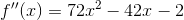.

Next, plug in x=1 to get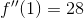.

The double derivative is a positive number, which means the concavity is Concave Up.

### Example Question #2 : Second Derivatives

Find the second derivative.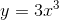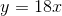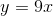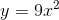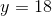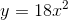Explanation:

We need to find the second derivative. To do this we will use power rule twice. We will take the first derivative by power rule, then the second derivative by power rule once again. Power rule says that we take the exponent of the “x” value and bring it to the front. Then we subtract one from the exponent.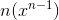Therefore, the first derivative is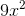and the second derivative is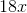by power rule.

### Example Question #1 : Relationship Between The Concavity Of ƒ And The Sign Of ƒ''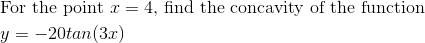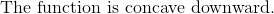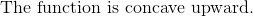Explanation: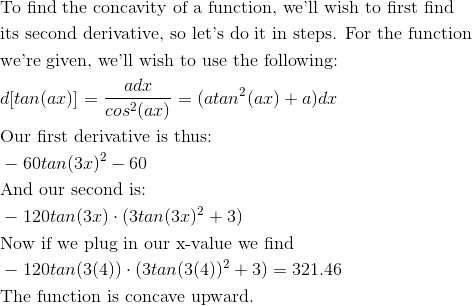### Example Question #2 : Relationship Between The Concavity Of ƒ And The Sign Of ƒ''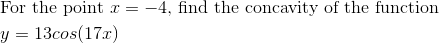Explanation: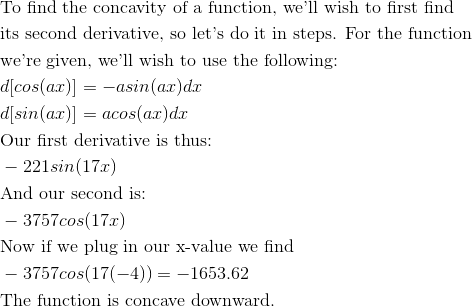### Example Question #3 : Relationship Between The Concavity Of ƒ And The Sign Of ƒ''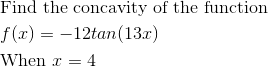Explanation: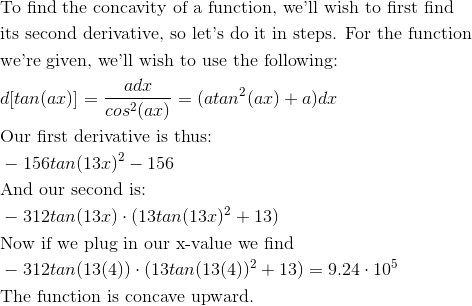### Example Question #6 : Second Derivatives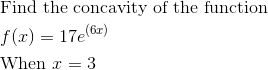Explanation: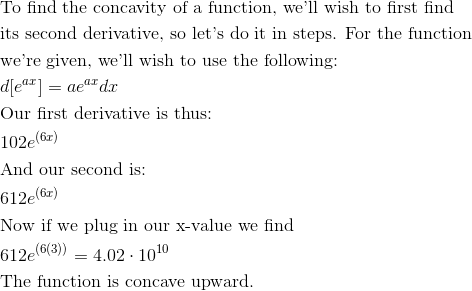### Example Question #2 : Relationship Between The Concavity Of ƒ And The Sign Of ƒ''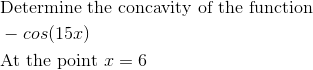Explanation: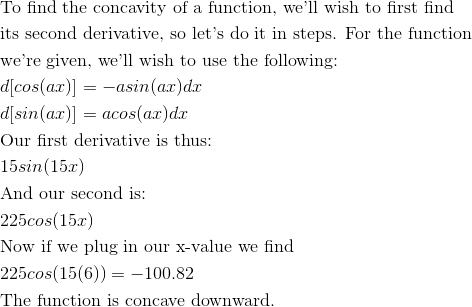### Example Question #4 : Relationship Between The Concavity Of ƒ And The Sign Of ƒ''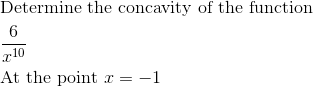Explanation: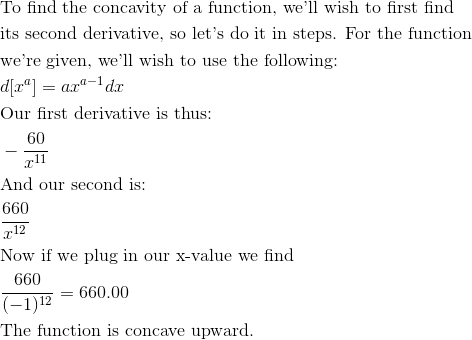### Example Question #2 : Second Derivatives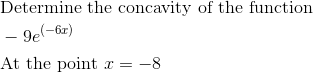Explanation: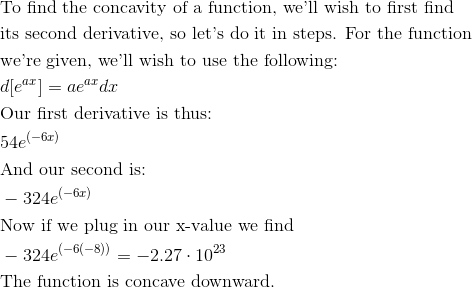### Example Question #4 : Relationship Between The Concavity Of ƒ And The Sign Of ƒ''